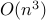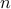# Optimal Solutions for Sparse Principal Component Analysis

• Authors: A. d'Aspremont, F. Bach, L. El Ghaoui.

• Status: Journal of Machine Learning Research, 9(Jul):1269–1294, 2008.

• Abstract: Given a sample covariance matrix, we examine the problem of maximizing the variance explained by a linear combination of the input variables while constraining the number of nonzero coefficients in this combination. This is known as sparse principal component analysis and has a wide array of applications in machine learning and engineering. We formulate a new semidefinite relaxation to this problem and derive a greedy algorithm that computes a full set of good solutions for all target numbers of non zero coefficients, with total complexity, whereis the number of variables. We then use the same relaxation to derive sufficient conditions for global optimality of a solution, which can be tested inper pattern. We discuss applications in subset selection and sparse recovery and show on artificial examples and biological data that our algorithm does provide globally optimal solutions in many cases.

• Bibtex reference:

```@article{ABE:08a,
Author = {A. d'Aspremont, F. Bach and L. {El Ghaoui}},
Journal = {Journal of Machine Learning Research},
Month = {July},
Pages = {1269--1294},
Title = {Optimal Solutions for Sparse Principal Component Analysis},
Volume = {9},
Year = {2008}}
```